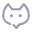# 格式化金额，每三位加个,

super
2020-11-19 08:35882

``````echo number_format(1024);
// 1,024
``````

``````echo number_format(1024.11, 2, '.', ',');
// 1,024.11``````

``````echo number_format(1024, 2, '.', ',');
// 1,024.00``````

## number_format

(PHP 4, PHP 5, PHP 7)

number_format — 以千位分隔符方式格式化一个数字

### 说明 ¶

number_format ( float `\$number` [, int `\$decimals` = 0 ] ) : string

number_format ( float `\$number` , int `\$decimals` = 0 , string `\$dec_point` = "." , string `\$thousands_sep` = "," ) : string

### 参数 ¶

`number`

`decimals`

`dec_point`

`thousands_sep`

0 条讨论